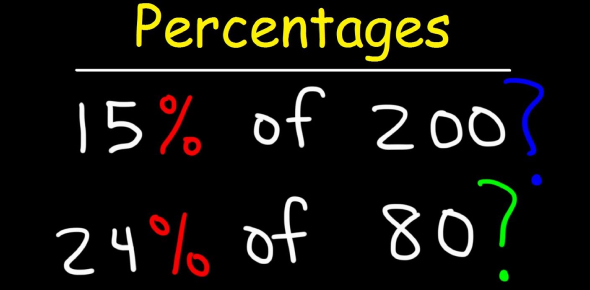# Percentage Quiz Questions And Answers

15 Questions | Attempts: 16987
ShareSettingsAre you quick at solving percentage questions? Can you pass this percentage quiz that we've created below for you? You see percentages all the time, whether it's on the resulting paper for an examination you took in school or college or as a review for a film or book, to let you know how good it is. What you might not know is how these percentages can be calculated if you need to get a certain value from a larger one. What is 15% of 660, for example? Play this quiz and find out.

• 1.
What is 10% of 210?
• A.

205

• B.

21

• C.

2.1

• D.

2

• E.

210

• 2.
What is 10% of 130?
• A.

13

• B.

1.3

• C.

26

• D.

13.5

• E.

130

• 3.
What is 10% of 365?
• A.

36

• B.

35

• C.

36.5

• D.

35.6

• E.

365

• 4.
What is 15% of 400?
• A.

40

• B.

20

• C.

60

• D.

50

• E.

44

• 5.
What is 15% of 660?
• A.

66

• B.

33

• C.

500

• D.

99

• E.

4

• 6.
What is 10% of 4000?
• A.

400

• B.

40

• C.

140

• D.

44

• 7.
What is 5% of 5000?
• A.

2500

• B.

250

• C.

2050

• D.

500

• 8.
What is 25% of 8000?
• A.

4000

• B.

2000

• C.

800

• D.

4400

• 9.
What is 12% of 9000?
• A.

1600

• B.

1800

• C.

1080

• D.

900

• 10.
What is 30% of 10,000?
• A.

100

• B.

3000

• C.

300

• D.

30000

• 11.
What is 23% of 5000?
• A.

1050

• B.

1150

• C.

5200

• D.

4670

• 12.
What is 18% of 5400?
• A.

9700

• B.

450

• C.

9720

• D.

972

• 13.
What will be 45% of 45000?
• A.

20500

• B.

20250

• C.

30000

• D.

4400

• 14.
What is 55% of 6500?
• A.

3500

• B.

3050

• C.

3575

• D.

4575

• 15.
What is 65% of 2300?
• A.

1400

• B.

1490

• C.

1495

• D.

1295

## Related TopicsBack to top
×

Wait!
Here's an interesting quiz for you.